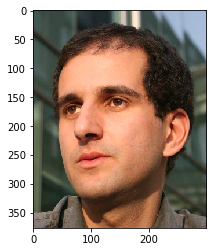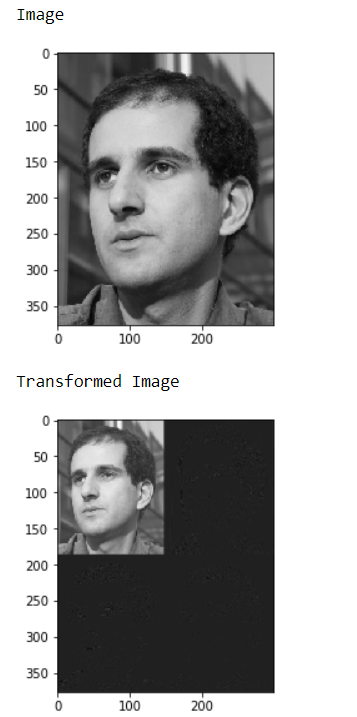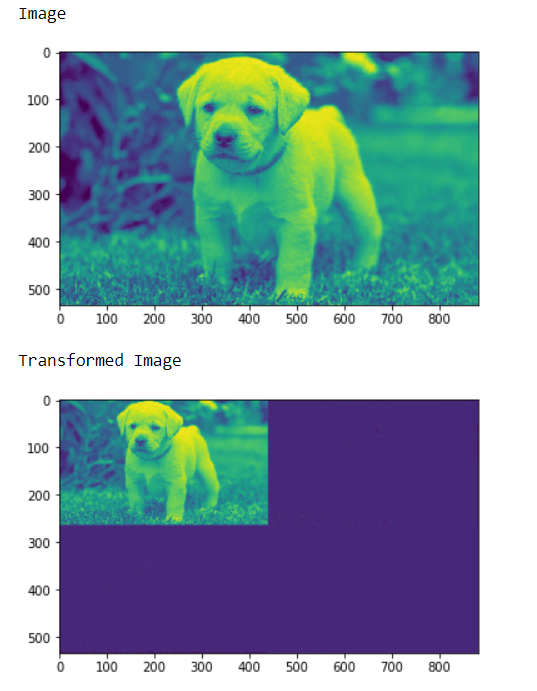# Mahotas – Transforming image using Daubechies wavelet

In this article we will see how we can transform image using daubechies wavelet in mahotas. In general the Daubechies wavelets are chosen to have the highest number A of vanishing moments, (this does not imply the best smoothness) for given support width 2A. There are two naming schemes in use, DN using the length or number of taps, and dbA referring to the number of vanishing moments. So D4 and db2 are the same wavelet transform.

In this tutorial we will use “luispedro” image, below is the command to load it.

`mahotas.demos.load('luispedro')`

Below is the luispedro imageIn order to do this we will use `mahotas.daubechies` method

Syntax : mahotas.daubechies(img, ‘D8’)

Argument : It takes image object and string i.e one of ‘D2’, ‘D4’, … ‘D20’ as argument

Return : It returns image object

Note : Input image should be filtered or should be loaded as grey

In order to filter the image we will take the image object which is numpy.ndarray and filter it with the help of indexing, below is the command to do this

`image = image[:, :, 0]`

Example 1:

 `# importing various libraries ` `import` `numpy as np ` `import` `mahotas ` `import` `mahotas.demos ` `from` `mahotas.thresholding ``import` `soft_threshold ` `from` `matplotlib ``import` `pyplot as plt ` `from` `os ``import` `path ` ` `  `# loading image ` `f ``=` `mahotas.demos.load(``'luispedro'``, as_grey ``=` `True``) ` ` `  `# making ply gray ` `plt.gray() ` ` `  `# showing image ` `print``(``"Image"``) ` `plt.imshow(f) ` `plt.show() ` ` `  `# Transform using D8 Wavelet to obtain transformed image t ` `t ``=` `mahotas.daubechies(f, ``'D8'``) ` ` `  `# showing transformed image ` `print``(``"Transformed Image"``) ` `plt.imshow(t) ` `plt.show() `

Output :Example 2:

 `# importing required libraries ` `import` `mahotas ` `import` `numpy as np ` `from` `pylab ``import` `imshow, show ` `import` `os ` ` `  ` `  `# loading iamge ` `img ``=` `mahotas.imread(``'dog_image.png'``) ` ` `  `# filtering iamge ` `img ``=` `img[:, :, ``0``] ` ` `  `# showing image ` `print``(``"Image"``) ` `imshow(img) ` `show() ` ` `  `     `  `# Transform using D8 Wavelet to obtain transformed image t ` `t ``=` `mahotas.daubechies(img, ``'D8'``) ` ` `  `# showing transformed image ` `print``(``"Transformed Image"``) ` `imshow(t) ` `show() `

Output :Attention geek! Strengthen your foundations with the Python Programming Foundation Course and learn the basics.

To begin with, your interview preparations Enhance your Data Structures concepts with the Python DS Course.

My Personal Notes arrow_drop_upCheck out this Author's contributed articles.

If you like GeeksforGeeks and would like to contribute, you can also write an article using contribute.geeksforgeeks.org or mail your article to contribute@geeksforgeeks.org. See your article appearing on the GeeksforGeeks main page and help other Geeks.

Please Improve this article if you find anything incorrect by clicking on the "Improve Article" button below.

Article Tags :

Be the First to upvote.

Please write to us at contribute@geeksforgeeks.org to report any issue with the above content.